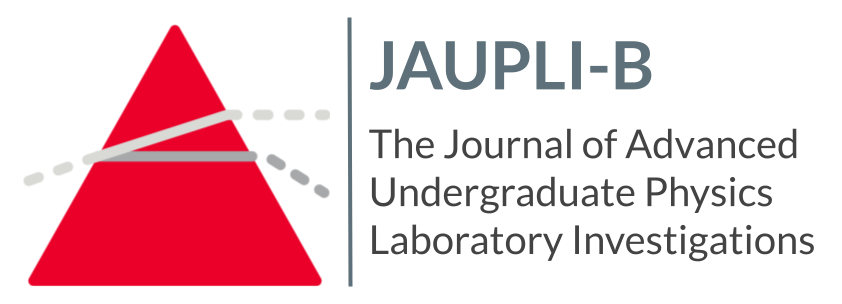•
•#### Title

Decay Time of a Damped Pendulum

#### Abstract

A number of labs have been conducted to find the equation for a damped pendulum. A damped pendulum's behavior will be analyzed and fit to this equation. This lab focused on tau, the decay constant. It was hypothesized that the decay constant would be greater with increasing initial angles, ultimately because of the pendulum's greater velocity. A pendulum was hung on a rotary motion sensor to detect its activity when released from different angles from the vertical. Its behavior was observed and fit to the equation for the behavior of a damped pendulum. It was found that decay time and initial angle are not directly proportional. This could be due to a changing frequency in the swing of the pendulum.

COinS